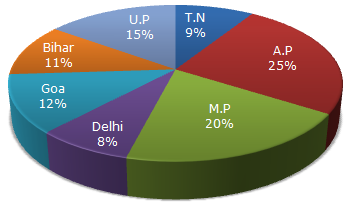# Data Interpretation - Pie Charts - Discussion

Study the following graph and the table and answer the questions given below.

Data of different states regarding population of states in the year 1998Total population of the given States = 3276000.

 States Sex and Literacy wise Population Ratio Sex Literacy M F Literate Illiterate A.P 5 3 2 7 M.P 3 1 1 4 Delhi 2 3 2 1 Goa 3 5 3 2 Bihar 3 4 4 1 U.P. 3 2 7 2 T.N. 3 4 9 4

2.

What was the total number of illiterate people in A.P. and M.P. in 1998?

 [A]. 876040 [B]. 932170 [C]. 981550 [D]. 1161160

Explanation:

 No. of illiterate people in A.P. =7 of (25% of 3276000)= 637000. 9

 No. of illiterate people in M.P. =4 of (20% of 3276000)= 524160. 5Total number = (637000 + 524160) = 1161160.

 Monika said: (Mar 3, 2012) How its value is 7/9 its value will be 7/7?

 Sheetal said: (Sep 8, 2012) How its value is 7/9 ?

 Abi said: (May 7, 2013) The value of illiterate in A.P and the total value of literate and illiterate in A.P must be added (i.e)illiterate = 7/literate+illiterate = 7+2 = 9. Hence it is 7/9.

 Ravindra Chavanke said: (May 22, 2014) How 7/9 is 25% and 4/5 is 20% of 3276000?

 Siddharth said: (Apr 16, 2016) Is there any shortcut method to solve this problem?